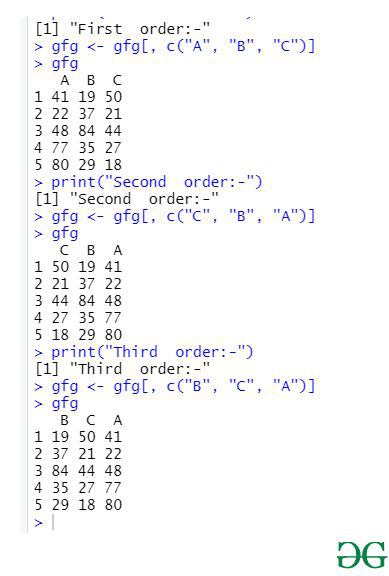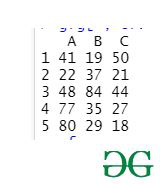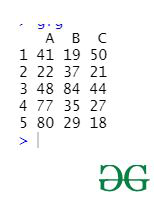# Reorder DataFrame by column name in R

• Last Updated : 05 Apr, 2021

It is very difficult any time taking task if we reorder the column name, so we use R Programming Language to do it effectively. In this article, we will be discussing the three different ways to reorder a given DataFrame by column name in R.

Method 1: Manually selecting the new order of the column names according to the user

In this particular method, the user has the choice to rearrange the columns name according to his/her own choice, In this method, the user just needs to give the order in which he/she want the column name to rearrange and then the columns name are reordered as per the given choice by the user.

Steps –

• Create dataframe
• Specify required order
• Apply this order to dataframe
• Display dataframe

Example:

## R

 `gfg = ``data.frame``(C = ``c``(50, 21, 44, 27, 18),``                 ``A = ``c``(41, 22, 48, 77, 80), ``                 ``B = ``c``(19, 37, 84, 35, 29))`` ` `print``(``"First  order:-"``)`` ` `gfg <- gfg[, ``c``(``"A"``, ``"B"``, ``"C"``)]``gfg`` ` `print``(``"Second  order:-"``)``gfg <- gfg[, ``c``(``"C"``, ``"B"``, ``"A"``)]``gfg`` ` `print``(``"Third  order:-"``)``gfg <- gfg[, ``c``(``"B"``, ``"C"``, ``"A"``)]``gfg`

Output:Method 2: Using order & names Functions

In this method of reordering the column name, we will be using, order and the names function which will sort the column names in the alphabetic order.

The order function used here returns the position of each element of its input in ascending or descending order.

Syntax:
order(x, decreasing, na.last)

Parameters:
x: Vector to be sorted
decreasing: Boolean value to sort in descending order
na.last: Boolean value to put NA at the end

Approach

• Create data frame
• Reorder alphabetically
• Display data frame

Example:

## R

 `gfg = ``data.frame``(C = ``c``(50, 21, 44, 27, 18),``                 ``A = ``c``(41, 22, 48, 77, 80),``                 ``B = ``c``(19, 37, 84, 35, 29))`` ` `gfg[ , ``order``(``names``(gfg))]`

Output:Method 3: Using dplyr Package:

To reorder the column name using dplyr package user must need to install and load the package using the given below syntax. With this method of reordering the columns name the column name get automatically get sorted in alphabetical order,

Syntax:

sort(name_of_vector, decreasing = TRUE)

Parameters:

name_of_vector: Vector to be sorted

decreasing: Boolean value to sort in descending order

Approach

• Create data frame
• Sort data using function
• Display frame

Example:

## R

 `gfg = ``data.frame``(C = ``c``(50, 21, 44, 27, 18),``                 ``A = ``c``(41, 22, 48, 77, 80),``                 ``B = ``c``(19, 37, 84, 35, 29))`` ` `gfg <- gfg %>%                      ``  ``select``(``sort``(``names``(.)))`` ` `gfg`

Output:My Personal Notes arrow_drop_up